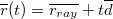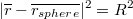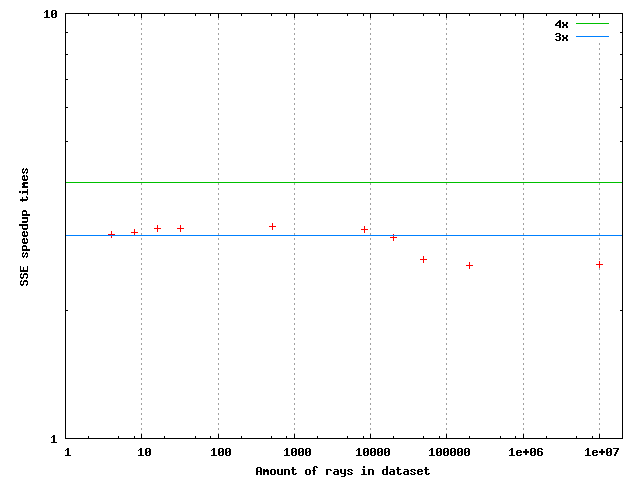Algorithm example 3 - Ray sphere intersection

Intersection test between a ray and a sphere will be an example of algebraic geometry. Inserting a general line equation:Into sphere surface equation:Gives a quadratic equation for t in terms of the known input vectors and R. In code the solution for t can be implemented as follows:

inline bool intersect(const vec3& raydir, const vec3& rayorig,const vec3& pos,
const float& rad, vec3& hitpoint,float& distance, vec3& normal)
{
float a = sum(raydir*raydir);
float b = sum(raydir * (2.0f * ( rayorig - pos)));
float D = b*b + (-4.0f)*a*c;

// If ray can not intersect then stop
if (D < 0)
return false;
D=sqrtf(D);

// Ray can intersect the sphere, solve the closer hitpoint
float t = (-0.5f)*(b+D)/a;
if (t > 0.0f)
{
distance=sqrtf(a)*t;
hitpoint=rayorig + t*raydir;
}
else
return false;
return true;

This can be vectorized for example by intersecting a packet of four rays against a single sphere. The other possibility is to intersect a single ray against four spheres. There is control logic in the code, which has to be replaced by mask operations. Additionally the vectorized intersection function will be taking a mask, which determines which rays should be touched by the function in the first place. An implementation follows:

inline vec4b intersect(const mat4x3& raydir, const mat4x3& rayorig, const vec3& pos,
{
vec4 raydirx = raydir;
vec4 raydiry = raydir;
vec4 raydirz = raydir;
vec4 a = raydirx*raydirx + raydiry*raydiry + raydirz*raydirz;
vec4 rayorigx = rayorig;
vec4 rayorigy = rayorig;
vec4 rayorigz = rayorig;
vec4 b = raydirx * 2.0f * (rayorigx - pos) +
raydiry * 2.0f * (rayorigy - pos) + raydirz * 2.0f * (rayorigz - pos);
vec4 c = sum(pos*pos) + rayorigx*rayorigx + rayorigy*rayorigy + rayorigz*rayorigz -

vec4 D = b*b + (-4.0f)*a*c;

D = Sqrt(D);

vec4 t = (-0.5f)*(b+D)/a;

// All rays hit
{
distance = Sqrt(a) * t;
vec4 hitpointx = rayorigx + t*raydirx;
vec4 hitpointy = rayorigy + t*raydiry;
vec4 hitpointz = rayorigz + t*raydirz;
hitpoint=hitpointx;
hitpoint=hitpointy;
hitpoint=hitpointz;
}
else // some rays hit
{
distance = Condition(mask, Sqrt(a) * t, distance);
vec4 hitpointx = rayorigx + t*raydirx;
vec4 hitpointy = rayorigy + t*raydiry;
vec4 hitpointz = rayorigz + t*raydirz;